Kids Encyclopedia Facts

In geometry, the radius of a circle or sphere is the shortest connection between the center and the boundary. It is half of the diameter.

r= d ÷ 2

d= 2 x r = d= r + r

The relationship between the radius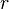$r$ and the circumference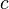$c$ of a circle is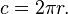$~c = 2\pi r.$
The area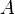$A$ of a circle of radius$r$ is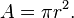$~A = \pi r^2.$